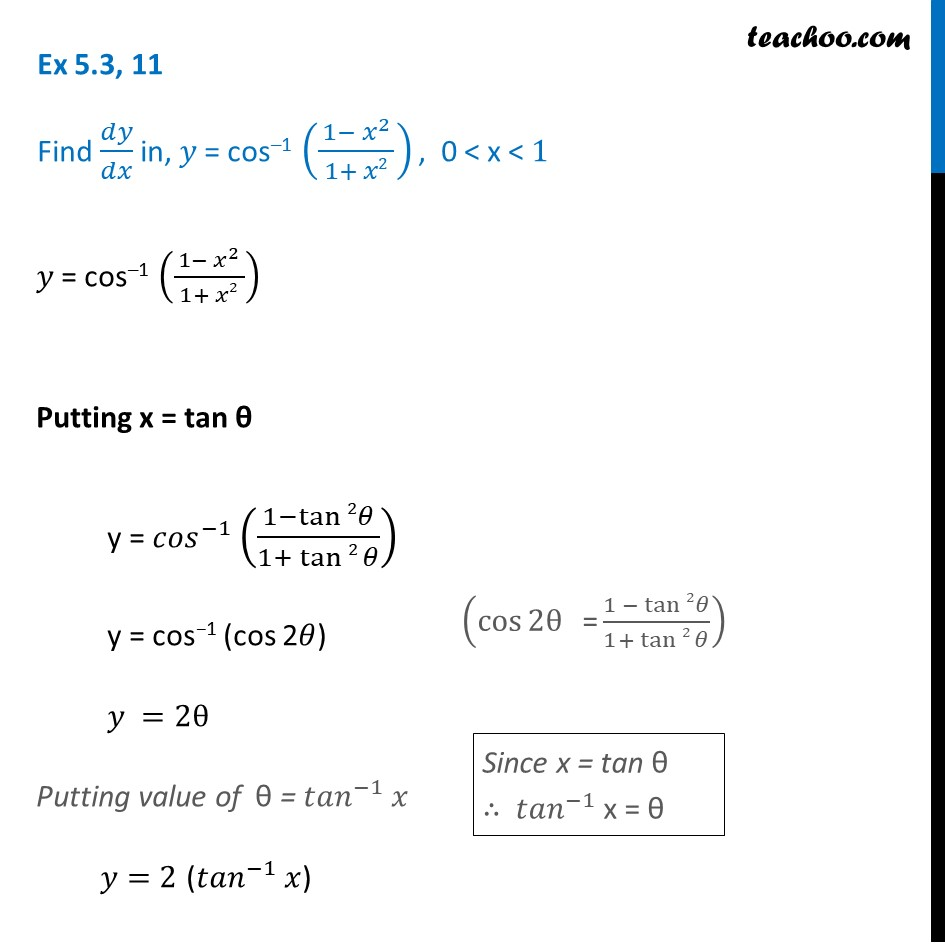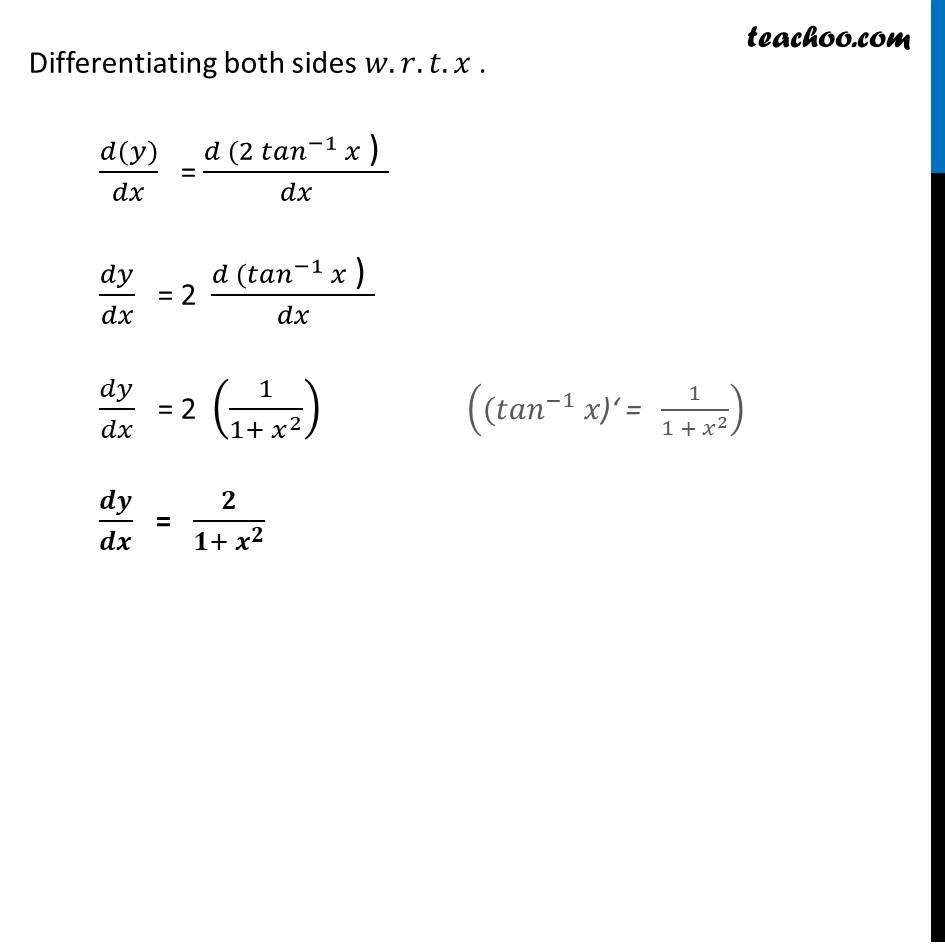Ex 5.3

Chapter 5 Class 12 Continuity and Differentiability
Serial order wiseLearn in your speed, with individual attention - Teachoo Maths 1-on-1 Class

### Transcript

Ex 5.3, 11 Find 𝑑𝑦/𝑑𝑥 in, 𝑦 = cos–1 ((1− 𝑥^2)/( 1+ 𝑥2 )) , 0 < x < 1 𝑦 = cos–1 ((1− 𝑥^2)/( 1+ 𝑥2 )) Putting x = tan θ y = 〖𝑐𝑜𝑠〗^(−1) ((1−tan⁡2 𝜃)/(1+ tan⁡2 𝜃)) y = cos−1 (cos 2𝜃) 𝑦 =2θ Putting value of θ = 〖𝑡𝑎𝑛〗^(−1) 𝑥 𝑦=2 (〖𝑡𝑎𝑛〗^(−1) 𝑥) (cos⁡2θ " =" (1 − tan⁡2 𝜃)/(1+ tan⁡2 𝜃)) Since x = tan θ ∴ 〖𝑡𝑎𝑛〗^(−1) x = θ